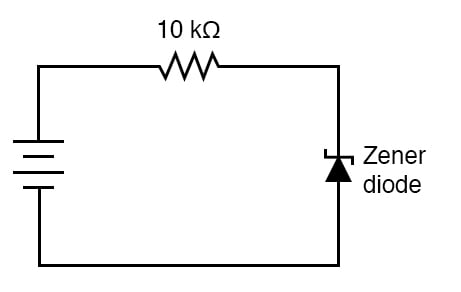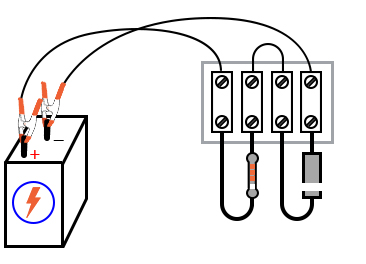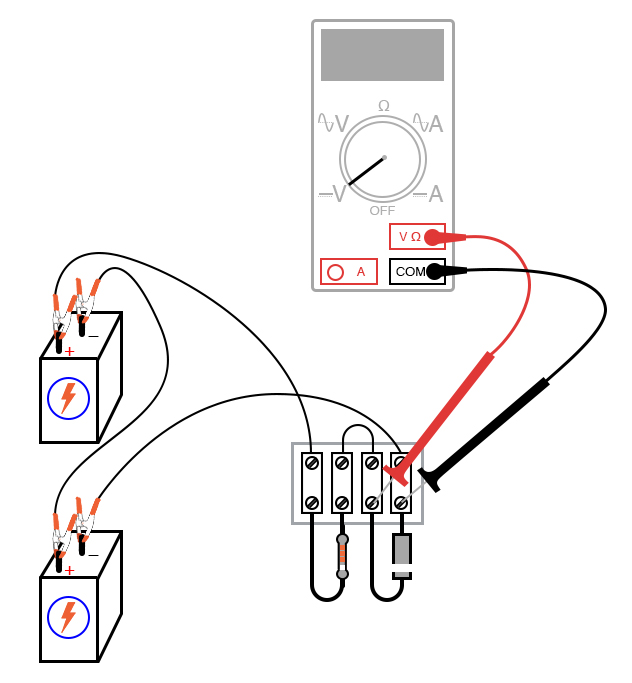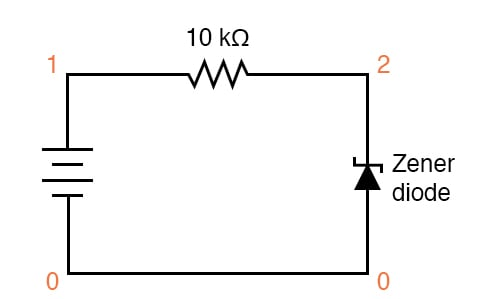# Voltage Regulator

## Chapter 5 - Discrete Semiconductor Circuits

PARTS AND MATERIALS

• Four 6 volt batteries
• Zener diode, 12 volt—type 1N4742 (Radio Shack catalog # 276-563 or equivalent)
• One 10 kΩ resistor

Any low-voltage zener diode is appropriate for this experiment. The 1N4742 model listed here (zener voltage = 12 volts) is but one suggestion.

Whatever diode model you choose, I highly recommend one with a zener voltage rating greater than the voltage of a single battery, for a maximum learning experience. It is important that you see how a zener diode functions when exposed to a voltage less than its breakdown rating.

CROSS-REFERENCES

Lessons In Electric Circuits, Volume 3, chapter 3: “Diodes and Rectifiers

LEARNING OBJECTIVES

• Zener diode function

SCHEMATIC DIAGRAMILLUSTRATIONINSTRUCTIONS

Build this simple circuit, being sure to connect the diode in “reverse-bias” fashion (cathode positive and anode negative), and measure the voltage across the diode with one battery as a power source. Record this voltage drop for future reference.

Also, measure and record the voltage drop across the 10 kΩ resistors. Modify the circuit by connecting two 6-volt batteries in series, for 12 volts total power source voltage. Re-measure the diode’s voltage drop, as well as the resistor’s voltage drop, with a voltmeter:Connect three, then four 6-volt batteries together in series, forming an 18 volt and 24-volt power source, respectively. Measure and record the diode’s and resistor’s voltage drops for each new power supply voltage.

What do you notice about the diode’s voltage drop for these four different source voltages? Do you see how the diode voltage never exceeds a level of 12 volts?

What do you notice about the resistor’s voltage drop for these four different source voltage levels? Zener diodes are frequently used as voltage regulating devices because they act to clamp the voltage drop across themselves at a predetermined level.

Whatever excess voltage is supplied by the power source becomes dropped across the series resistor. However, it is important to note that a zener diode cannot make up for a deficiency in source voltage.

For instance, this 12-volt zener diode does not drop 12 volts when the power source is only 6 volts strong. It is helpful to think of a zener diode as a voltage limiter: establishing a maximum voltage drop, but not a minimum voltage drop.

COMPUTER SIMULATION

Schematic with SPICE node numbers:Netlist (make a text file containing the following text, verbatim):

Zener diode v1 1 0 r1 1 2 10k d1 0 2 mod1 .model mod1 d bv=12 .dc v1 18 18 1 .print dc v(2,0) .end

A zener diode may be simulated in SPICE with a normal diode, the reverse breakdown parameter (bv=12) set to the desired zener breakdown voltage.

RELATED WORKSHEETS:

• Share
Published under the terms and conditions of the Design Science License